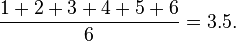CATEGORIES:

# The law of large Numbers

In probability theory, the law of large numbers (LLN) is a theorem that describes the result of performing the same experiment a large number of times. According to the law, the average of the results obtained from a large number of trials should be close to the expected value, and will tend to become closer as more trials are performed.

The LLN is important because it "guarantees" stable long-term results for the averages of some random events. For example, while a casino may lose money in a single spin of the roulette wheel, its earnings will tend towards a predictable percentage over a large number of spins. Any winning streak by a player will eventually be overcome by the parameters of the game. It is important to remember that the LLN only applies (as the name indicates) when a large number of observations are considered. There is no principle that a small number of observations will coincide with the expected value or that a streak of one value will immediately be "balanced" by the others (see the gambler's fallacy).

Examples

For example, a single roll of a fair six-sided die produces one of the numbers 1, 2, 3, 4, 5, or 6, each with equal probability. Therefore, the expected value of a single die roll isAccording to the law of large numbers, if a large number of six-sided dice are rolled, the average of their values (sometimes called the sample mean) is likely to be close to 3.5, with the precision increasing as more dice are rolled.

It follows from the law of large numbers that the empirical probability of success in a series of Bernoulli trials will converge to the theoretical probability. For a Bernoulli random variable, the expected value is the theoretical probability of success, and the average of n such variables (assuming they are independent and identically distributed (i.i.d.)) is precisely the relative frequency.

For example, a fair coin toss is a Bernoulli trial. When a fair coin is flipped once, the theoretical probability that the outcome will be heads is equal to 1/2. Therefore, according to the law of large numbers, the proportion of heads in a "large" number of coin flips "should be" roughly 1/2. In particular, the proportion of heads after n flips will almost surely converge to 1/2 as n approaches infinity.

Though the proportion of heads (and tails) approaches 1/2, almost surely the absolute (nominal) difference in the number of heads and tails will become large as the number of flips becomes large. That is, the probability that the absolute difference is a small number, approaches zero as the number of flips becomes large. Also, almost surely the ratio of the absolute difference to the number of flips will approach zero. Intuitively, expected absolute difference grows, but at a slower rate than the number of flips, as the number of flips grows.

History

The Italian mathematician Gerolamo Cardano

(1501–1576) stated without proof that the accuracies of empirical statistics tend to improve with the number of trials. This was then formalized as a law of large numbers. A special form of the LLN (for a binary random variable) was first proved by Jacob Bernoulli. It took him over 20 years to develop a sufficiently rigorous mathematical proof which was published in his Ars Conjectandi (The Art of Conjecturing) in 1713. He named this his "Golden Theorem" but it became generally known as "Bernoulli's Theorem". This should not be confused with the principle in physics with the same name, named after Jacob Bernoulli's nephew Daniel Bernoulli. In 1837, S.D. Poisson further described it under the name "la loi des grands nombres" ("The law of large numbers"). Thereafter, it was known under both names, but the "Law of large numbers" is most frequently used.

Date: 2015-01-11; view: 1214

 <== previous page | next page ==> Vegetation and Wildlife | The letter heading and the layout
doclecture.net - lectures - 2014-2023 year. Copyright infringement or personal data (0.011 sec.)